Estimation of The Drag of a Roof Mounted Antenna (Ford AU Falcon)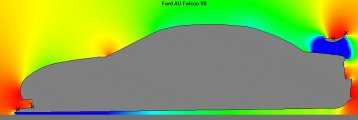1. Introduction

With the increasing use of telemetry and in-car radio communication in motorsport, a variety of radio antennas are now being fitted to racing cars. This report will consider the potential aerodynamic impact of a radio antenna mounted on the roof of a Ford AU Falcon V8 touring car. The AU Falcon was chosen as geometric data for the car body profile was available to us.

The particular case being considered here is for a typical V8 Supercar. These vehicles tend to operate with two antennas mounted on the roof. There is usually a larger main antenna mounted in the centre of the roof panel and a smaller antenna mounted half to one metre behind the main antenna. Previously the main antenna was about 300 mm in length and quite rigid, but there now appears to be a move toward using a much longer, more flexible component.

As a general comment, placing an antenna in the centre of a car's roof panel represents a very favourable location as far as the performance of the antenna is concerned with respect to radio communication, but this location would be the worst possible location aerodynamically. The air flowing over a car roof is travelling faster than the speed of the car itself. Aerodynamic drag varies with the square of velocity, so the current antenna location is possibly one of the highest drag locations on the whole car body.

2. Theory

The underlying theory for this analysis relates to the behaviour of a cylinder in an air flow. The amount of drag generated by a cylinder will vary greatly depending on the relative size of the cylinder and the speed of the air.

These values are related by using a parameter called Reynold's Number (Re). This is a dimensionless value which correlates fluid behavior for different object sizes and flow speeds. Regardless of an object's physical size and the speed of the air flowing past it, if two objects have a similar Reynold's Number then the nature of the flow around them will be very similar.

The behaviour of a cylinder in an air flow will vary greatly with the Reynold's Number. It has been determined experimentally that below a Reynold's Number of 1.5 x 10^5 you have what is called "sub-critical" flow, and a typical cylinder will have a drag coefficient value of about 1.20, while above a Reynold's Number of 4 x 10^5 you will have what is called "super-critical" flow, with a drag coefficient value of about 0.40. Between these ranges there is a transition region. The simple relevance of this is that in "super-critical" flow the drag of the cylinder will be one-third that of the same cylinder operating in "sub-critical" flow.

This situation is not dissimilar to that of the golf ball. A golf ball with a smooth surface was found to operate within a certain Reynold's Number region, with a particular drag. By applying a rough surface to the golf ball (the well known golf ball dimple pattern) it was found the flow around the ball changed, and so operated in a region with lower drag. The nett result was better performance through achieving this lower drag level.

The reason for the variation in drag between “sub-critical” and “super-critical” flow relates to the wake generated when the air flows past a cylinder. The nature of the wake changes as the Reynold's Number increases. This involves the mix of laminar and turbulent flow of the passing air, and the point on the cylinder's surface where the laminar flow changes to turbulent flow.

Another feature to be considered is what is termed a "Karman Vortex Street". Under certain conditions vortices can be shed by the flow passing a cylinder. These vortices are generally shed in an ordered manner, from each side of the cylinder's surface, in turn. The nett result of this is that an oscillation can be set up, caused by these fluctuating forces on the cylinder. This process is the basis for wires sometimes "singing" when acted on by a wind of an appropriate velocity.

For the calculation of Reynold's Number and drag for a cylinder we base the cylinder size on its diameter, and its reference area (for drag calculations) on its frontal area (length x diameter).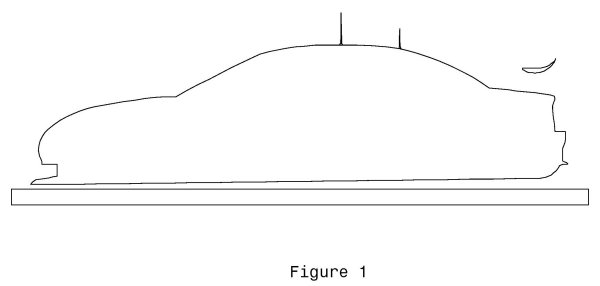3. Analysis

We can now perform a a theoretical analysis in order to estimate the likely drag of an antenna mounted on the car roof. A general view of the Falcon centreline body profile, and the typical antenna locations, is shown in Figure 1.

A racing car does not usually operate at a single speed, but over a speed range. We have assumed a speed range here from 128 km/h (80 mph), as the low end, up to 272 km/h (170 mph), as the high end, with 200 km/h (125 mph) as the average. We will perform calculations for all three speeds, so we can see how the various parameters may vary over this speed range.

We also need to define a typical antenna geometry, or two in this analysis. We will first consider a shorter, rigid antenna, typical of the older style. We will assume here an antenna length of 300 mm and a diameter of 8 mm, with a circular cross-section (as shown in Figure 1).

The Reynold's Number can be calculated, for velocity in metres per second and the reference length in metres, using the following equation;

 Re = (velocity x length) / 1.456 x 10^-5

Here we have a reference length of 0.008 metres (the diameter of the antenna). Using these values we can calculate the following Reynold's Numbers for the speed range being considered;

 80 mph = 128 km/h = 35.5 m/sec giving Re = 1.95 x 10^4 125 mph = 200 km/h = 55.5 m/sec giving Re = 3.05 x 10^4 170 mph = 272 km/h = 75.5 m/sec giving Re = 4.15 x 10^4

We can now consider a second case of an antenna twice as long (600 mm) but with only half the diameter (4 mm). This is intended to represent the more modern type of "whip" antenna now being used (as shown in Figure 3, Item A).

As before, for the Reynold’s Number calculation, our reference length is now 0.004 metres and we obtain;

 80 mph = 128 km/h = 35.5 m/sec giving Re = 9.75 x 10^3 125 mph = 200 km/h = 55.5 m/sec giving Re = 1.52 x 10^4 170 mph = 272 km/h = 75.5 m/sec giving Re = 2.07 x 10^4

For both the geometries considered here the effective Reynold's Number range is between 1.0 x 10^4 and 4.0 x 10^4, which is well into the "sub-critical" drag range for a circular cross-section. For Reynold's Numbers below 1.0 x 10^5 the drag coefficient for a cylinder is around 1.10 to 1.20 (depending on the particular reference source consulted).

From this we can now make an estimate of the aerodynamic drag. Aerodynamic drag is given by the equation;

 Drag = Cd x S x 0.5 x rho x Velocity^2

where "Cd" is the drag coefficient (Cd being 1.10 here), "S" is the reference area (0.3 x 0.008 = 0.6 x 0.004 = 0.0024 square metres, and the same for both geometries being considered) and "rho" is the air mass density (1.225 kg/m^3). From this we can now estimate the following drag values;

 80 mph = 128 km/h = 35.5 m/sec giving Drag = 2.04 Newtons 125 mph = 200 km/h = 55.5 m/sec giving Drag = 4.98 Newtons 170 mph = 272 km/h = 75.5 m/sec giving Drag = 9.22 Newtons

Oscillation Frequency

It is also possible to estimate the possible oscillation frequency of the antenna. This can be calculated using the equation;

 frequency = (velocity x SN) / length

where the velocity is in metres per second and the length is the reference length (diameter of the antenna) measured in metres. The value SN is termed the Strouhal Number, and for the flow conditions here it has an approximate value of 0.185 (obtained from graphs of experimental data).

From this we find the 8 mm diameter antenna has an oscillation frequency of 821 hertz at 35.5 m/sec (128 km/h), increasing to 1746 hertz at 75.5 m/sec (272 km/h). Similarly, the 4 mm diameter antenna has an oscillation frequency ranging from 1642 hertz to 3492 hertz.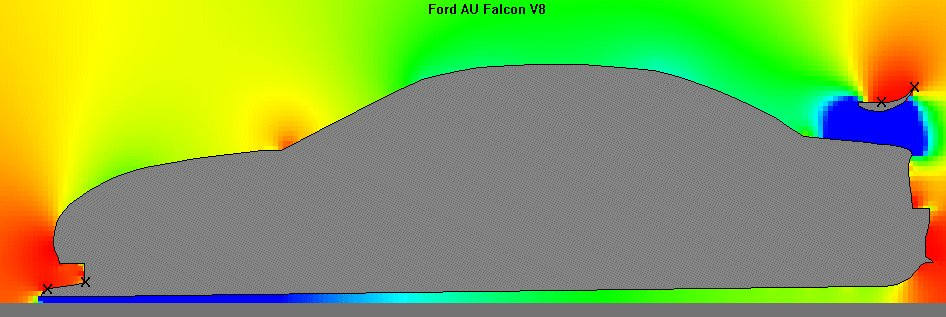Pressure Distribution

As a step in better understanding the airflow and pressure distribution around the vehicle body, the body centreline profile was entered into a 2D computational fluid dynamic analysis program (SUB-2D). The results of this analysis are shown in Figure 2.

The key point arising from this analysis is that the local airflow over the roof of the car has a much higher velocity than the freestream velocity. This was determined theoretically as being 38 percent higher, though in reality this may be more like 25 percent, as the theoretical analysis did not take into account viscous effects and other real-World interactions.

On this basis, for our drag calculation of air passing the antenna at 75.5 metres per second (272 km/h), the actual vehicle speed would have been slower, as the airflow over the roof is a locally accelerated flow with a value above the vehicle's road speed, by about 25 percent. Therefore the vehicle would probably have been travelling at about 60.5 m/sec (218 km/h) in this situation.

4. Conclusions

The key question arising out of this report is, just how important is drag reduction in optimising the performance of a racing car? The aerodynamic drag of the antenna is quite small, but it is a clear source of energy loss. There are a variety of ways in which this drag can be reduced, but to achieve this will involve some cost and effort. The amount of effort will also inevitably be a trade-off between the required radio performance and the reduction of aerodynamic drag.

The actual antenna installations are likely to have much higher drag than that calculated here. This analysis only estimated the drag of the central antenna rod. Real antenna installations involve a mounting bracket on the car roof and, in some cases, a thicker fitting part way along the antenna itself. Most cars also have a second, smaller antenna mounted behind the main unit. On this basis a real installation may have up to four times the drag calculated here. The actual drag will vary between cars as the antenna mounting fixtures can vary widely in their shape and location.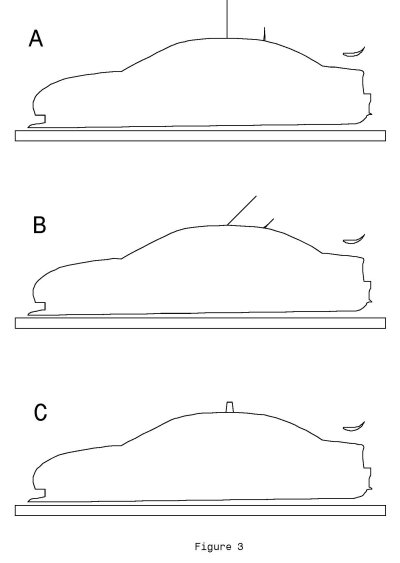5. Recommendations

There are a number of options available which could reduce the drag of a roof mounted antenna. It's important to note that the suggestions here are aimed at reducing aerodynamic drag but take no account of the effect on antenna performance as it relates to radio reception or transmission.

Perhaps the simplest option is to rake the antenna back at an angle (see Figure 3, Item B). As the angle is increased the effective cross-section of the antenna changes from a circle to an ellipse of increasing fineness. The drag coefficient of an ellipse is lower than for a circle of similar width, so this would provide a small aerodynamic advantage for minimal effort.

A second option would be to streamline the antenna rod. Changing the antenna from a circular cross-section to a streamline cross-section (a teardrop shape having the same width as the circular antenna, and a thickness-to-chord ratio of 25 percent) would reduce the drag coefficient from a value of about 1.10 to a value of about 0.70 (about a 35 percent drag reduction).

Changes to the cross-section other than by using a streamlined profile can also be usefully employed. Several options are presented in Figure 4.

Changing the antenna from a long thin cylinder to a broad plate is also a possible option (Figure 3, Item C), assuming the radio performance is not adversely affected by this arrangement.

In this case we can estimate the drag of such a plate, representing some theoretical antenna, 140 mm in height and 70 mm in width (the direction aligned with the airflow). We can assume an 11 percent thick aerofoil type cross-section, which, at a velocity of 75.5 m/sec (272 km/h) would have a Reynold's Number of 3.63 x 10^5, giving a drag coefficient (based on the planform area) of 0.10. The planform area would be;

 0.07 x 0.14 = 0.0098 square metres

Calculating the drag of this item gives us a value of 3.42 Newtons, compared to the 300 mm/600 mm antenna drag, at the same speed, of 9.22 Newtons. This example has been included primarily to highlight how a properly streamlined profile can offer dramatically lower drag than a circular cross-section, even if it has a much greater surface area.

There is also the potential to reduce drag by mounting the antenna on a different location on the car body. The roof is a location where the local air speed is quite a bit higher (a low pressure region) than that of the free stream airflow surrounding the car. Moving the antenna to the rear edge of the bonnet, where the local air velocity is slower (a higher pressure region), or to the boot lid, where the airflow is very turbulent and disturbed, should result in a measurable drag reduction. The disadvantage of this approach is that the radio signal may be blanketed from the antenna for some directions.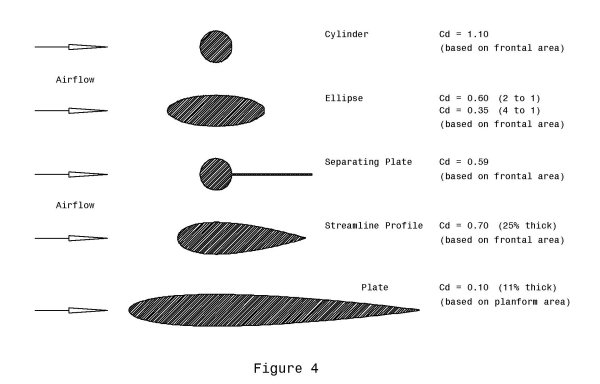6. References

 (1) Fluid Dynamic Drag - S F Hoerner Published by the author  1965 (2) Aerodynamics, Aeronautics & Flight Mechanics Barnes W McCormick John Wiley & Sons 1979 (3) Further Aerodynamics for Engineering Students Houghton & Boswell Edward Arnold Ltd 1969 (4) Pratt & Whitney Aeronautical Vest-Pocket Handbook Eighteenth Edition June 1978 (5) SUB-2D for Windows Version 1.06 SoftwAeronautics, Inc
 This report was prepared by Temporal Images in support of a development project being undertaken by presspley sports design. September 2005